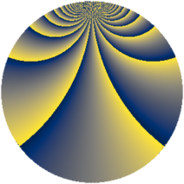# Properties

 Label 2736.3.ftLevel $2736$ Weight $3$ Character orbit 2736.ft Rep. character $\chi_{2736}(143,\cdot)$ Character field $\Q(\zeta_{18})$ Dimension $480$ Sturm bound $1440$

# Related objects

## Defining parameters

 Level: $$N$$ $$=$$ $$2736 = 2^{4} \cdot 3^{2} \cdot 19$$ Weight: $$k$$ $$=$$ $$3$$ Character orbit: $$[\chi]$$ $$=$$ 2736.ft (of order $$18$$ and degree $$6$$) Character conductor: $$\operatorname{cond}(\chi)$$ $$=$$ $$228$$ Character field: $$\Q(\zeta_{18})$$ Sturm bound: $$1440$$

## Dimensions

The following table gives the dimensions of various subspaces of $$M_{3}(2736, [\chi])$$.

Total New Old
Modular forms 5904 480 5424
Cusp forms 5616 480 5136
Eisenstein series 288 0 288

## Trace form

 $$480q + O(q^{10})$$ $$480q + 48q^{13} + 1680q^{49} + 672q^{61} + 240q^{73} + 288q^{85} + O(q^{100})$$

## Decomposition of $$S_{3}^{\mathrm{new}}(2736, [\chi])$$ into newform subspaces

The newforms in this space have not yet been added to the LMFDB.

## Decomposition of $$S_{3}^{\mathrm{old}}(2736, [\chi])$$ into lower level spaces

$$S_{3}^{\mathrm{old}}(2736, [\chi]) \cong$$ $$S_{3}^{\mathrm{new}}(228, [\chi])$$$$^{\oplus 6}$$$$\oplus$$$$S_{3}^{\mathrm{new}}(684, [\chi])$$$$^{\oplus 3}$$$$\oplus$$$$S_{3}^{\mathrm{new}}(912, [\chi])$$$$^{\oplus 2}$$